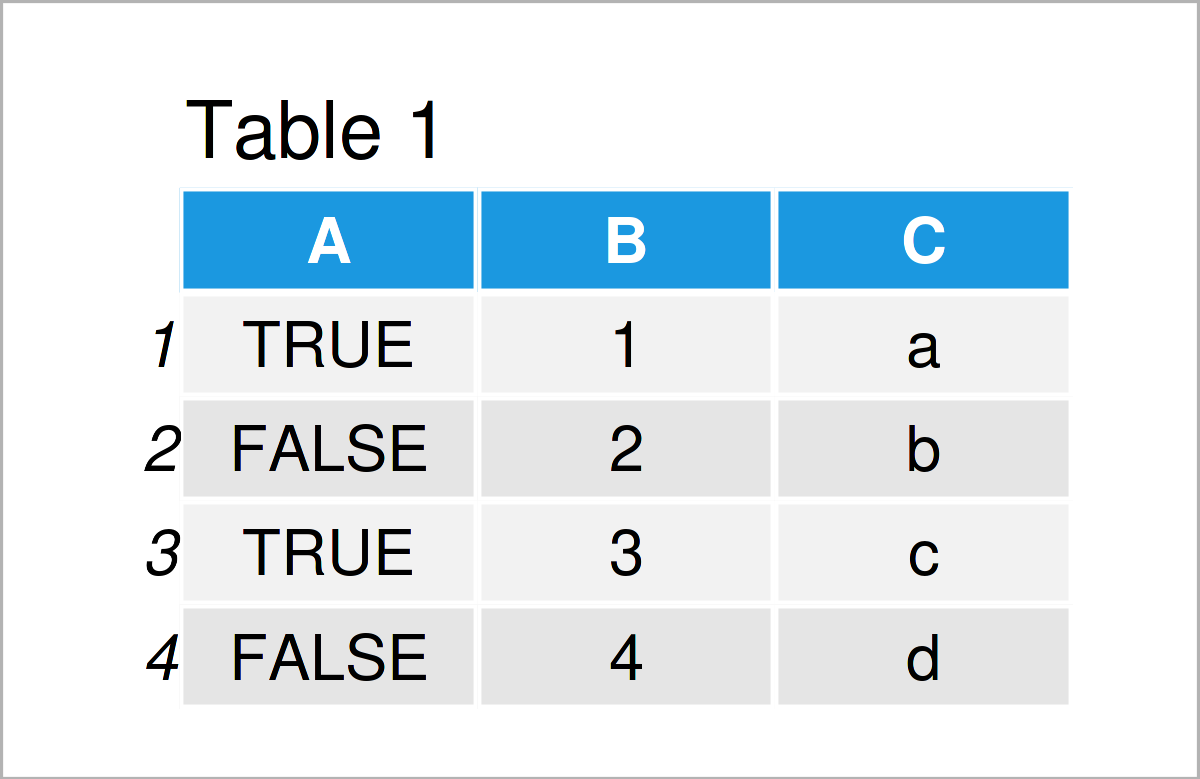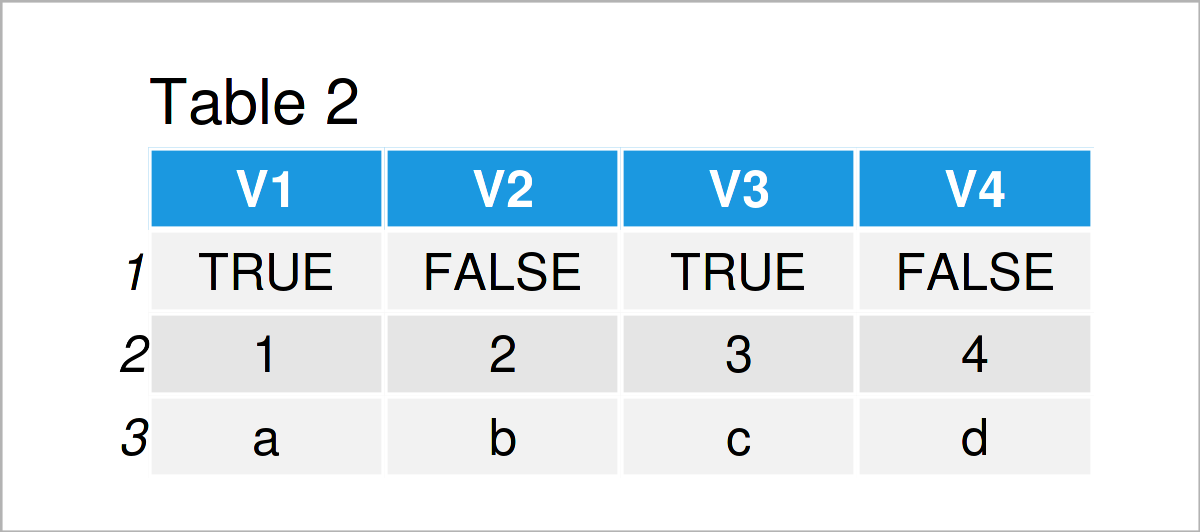# Transpose data.table in R (Example)

In this tutorial, you’ll learn how to switch the row and column indices of a data.table in R.

The tutorial will consist of the following topics:

## Example Data & Software Packages

To use the functions of the data.table package, we first have to install and load data.table.

```install.packages("data.table")            # Install data.table package

As a next step, let’s also create some example data:

```data_1 <- data.table(A = c(TRUE, FALSE, TRUE, FALSE),
B = 1:4,
C = letters[1:4])    # Create data.table
data_1                                    # Print data```As you can see based on Table 1, our example data is a data.table constituted of four rows and three columns.

## Example: Transpose the Example Data

In this example, I’ll illustrate how to transpose data_1. That is, we want to rotate the data such that the columns switch to the rows and vice versa.

To achieve this, we can use the transpose function as shown below:

```data_1_tr <- transpose(data_1)            # Transpose the data
data_1_tr```Table 2 shows the results of function transpose, which is from the data.table package. Before, we had a dataset with four rows and three columns. Now, we have a dataset with three rows and four columns.

## Video & Further Resources

You can find the CRAN page of the data.table package here and the github site here. Furthermore, you can find our introduction to the data.table package here.

I have recently published a video on my YouTube channel, which illustrates the R codes of this tutorial. You can find the video below.

In addition, you may want to read the other articles on my website.

To summarize: In this post, you have learned how to flip a data.table over its diagonal in the R programming language. In case you have additional questions or comments, please let me know in the comments.

This page was created in collaboration with Anna-Lena Wölwer. Have a look at Anna-Lena’s author page to receive further information about her academic background and the other articles she has written for Statistics Globe.

Subscribe to the Statistics Globe Newsletter